# rticks

Set or query r-axis tick values

## Syntax

``rticks(ticks)``
``rt = rticks``
``rticks('auto')``
``rticks('manual')``
``m = rticks('mode')``
``___ = rticks(pax,___)``

## Description

example

````rticks(ticks)` sets the r-axis tick values, which are the locations along the r-axis where the tick marks and grid lines appear. Specify `ticks` as a vector of increasing values; for example, `[0 2 4 6]`. This command affects the current axes.```
````rt = rticks` returns the current r-axis tick values as a vector.```

example

````rticks('auto')` sets an automatic mode, enabling the axes to determine the r-axis tick values. Use this option if you change the tick values and then want to set them back to the default values. ```
````rticks('manual')` sets a manual mode, freezing the r-axis tick values at the current values. Use this option if you want to retain the current tick values when resizing the axes or adding new data to the axes.```
````m = rticks('mode')` returns the current r-axis tick labels mode, which is either `'auto'` or `'manual'`. By default, the mode is automatic unless you specify tick values or change the mode to manual.```

example

````___ = rticks(pax,___)` uses the axes object `pax` instead of the current axes. Specify `pax` as the first input argument for any of the previous syntaxes.```

## Examples

collapse all

Create a polar plot. Display tick marks and grid lines along the r-axis at the values 0.1, 0.3, and 0.5. Then, specify a label for each tick mark.

```theta = linspace(0,2*pi); rho = theta/10; polarplot(theta,rho) rticks([0.1 0.25 0.5]) rticklabels({'r = .1','r = .3','r = .5'})```Display tick marks and grid lines along the r-axis at nonuniform values between 0 and 10. MATLAB® labels the tick marks with the numeric values.

```theta = 0:0.01:2*pi; rho = 10*sin(2*theta); polarplot(theta,rho) rticks([0 4 5 6 10])```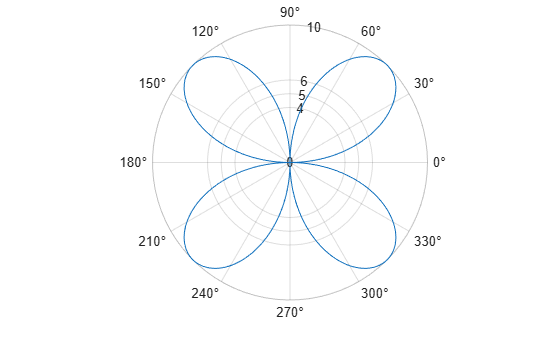Display tick marks and grid lines along the r-axis at increments of 10, starting from 0 and ending at 50.

```theta = 0:0.01:2*pi; rho = 50*sin(2*theta); polarplot(theta,rho) rticks(0:10:50)```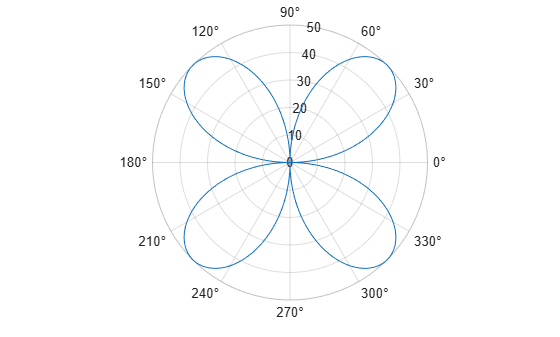Create a polar plot and specify the r-axis tick values. Then, set the r-axis tick values back to the default values.

```polarplot(1:10) rticks([0 5 10])````rticks('auto')`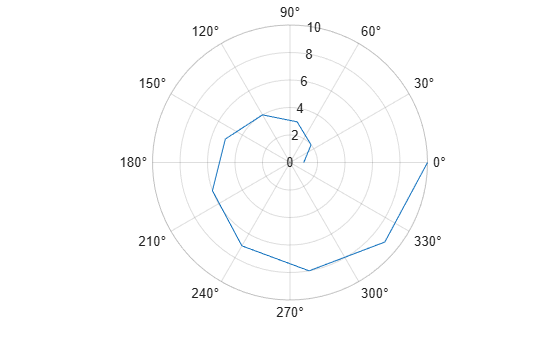Create polar axes and return the polar axes object `pax`. Ensure that `rticks` affects the polar axes you just created by passing `pax` as the first input argument to the function.

```pax = polaraxes; rticks(pax,[0 .5 1])```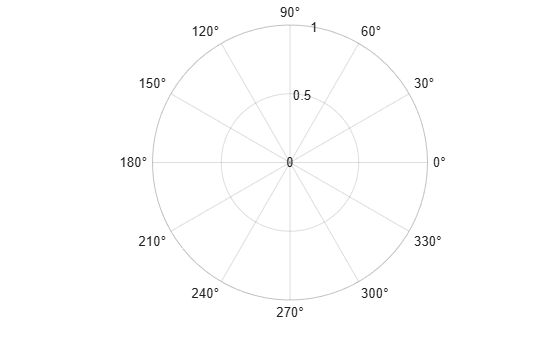Remove the tick marks and grid lines along the r-axis by specifying the tick values as an empty array.

```polarplot(1:100) rticks([])```## Input Arguments

collapse all

Tick values, specified as a vector of increasing values. If you do not want tick marks along the r-axis, specify an empty vector `[]`.

Example: `rticks([0 1 2 3 4])`

Example: `rticks(0:10:100)`

Example: `rticks([])`

Note

To specify the tick labels, use the `rticklabels` function.

Polar axes, or an array of polar axes. If you do not specify this argument, then `rticks` modifies the current axes (provided that the current axes is a polar axes object).

## Output Arguments

collapse all

Current tick values, returned as a vector.

Current mode, returned as one of these values:

• `'auto'` — Axes automatically determines the r-axis tick values.

• `'manual'` — Axes uses manually specified r-axis tick values.

collapse all

### Tick Values

The tick values are the locations along the r-axis where the tick marks appear. The tick labels are the labels that you see next to each tick mark. Set the tick values using the `rticks` function. Set the corresponding tick labels using the `rticklabels` function.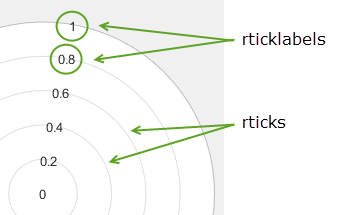## Algorithms

The `rticks` function sets and queries several axes properties related to the r-axis tick values.

• `RTick` — Property that stores the r-axis tick values.

• `RTickMode` — Property that stores the r-axis tick value mode. When you set the r-axis tick values, this property changes to `'manual'`.

## Version History

Introduced in R2016b